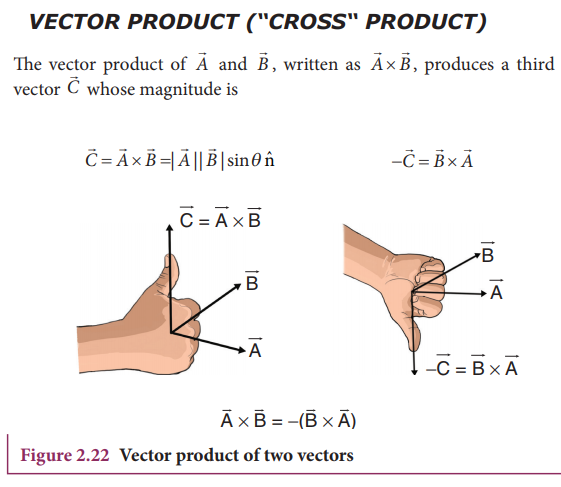Home | | Physics 11th std | The Vector Product of Two Vectors

# The Vector Product of Two Vectors

The vector product or cross product of two vectors is defined as another vector having a magnitude equal to the product of the magnitudes of two vectors and the sine of the angle between them.

The Vector Product of Two Vectors

Definition

The vector product or cross product of two vectors is defined as another vector having a magnitude equal to the product of the magnitudes of two vectors and the sine of the angle between them. The direction of the product vector is perpendicular to the plane containing the two vectors, in accordance with the right hand screw rule or right hand thumb rule (Figure 2.22).Properties of vector (cross) product.

A number of quantities used in Physics are defined through vector products. Particularly physical quantities representing rotational effects like torque, angular momentum, are defined through vector products.## Solved Example Problems for The Vector Product of Two VectorsTags : Definition, Properties, Solved Example Problems , 11th Physics : UNIT 2 : Kinematics
Study Material, Lecturing Notes, Assignment, Reference, Wiki description explanation, brief detail
11th Physics : UNIT 2 : Kinematics : The Vector Product of Two Vectors | Definition, Properties, Solved Example Problems